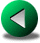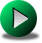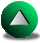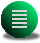Previous: The Munk Problem in
Next: Finite Difference Methods in
Up: Testing the Different Numerical

# Conclusions

We have tested in this chapter different FD, FE and SE methods. We first rule out the possibility of using a high order A-grid FD model because, in the presence of irregular geometries and for an inviscid flow, the effective truncation order is less than second order accurate. This was demonstrated in a circular domain and is due to the presence of steps occurring along the boundary when discretizing complex domains on Cartesian grids. The order of the model may have been preserved in curvilinear geometry, but we did not consider curvilinear grids as they are limited to smooth domains. The same applies to the second order C-grid model, although the loss of accuracy is less severe.

We also considered FE methods, some of which are quite simple (equal-order formulation). They all use linear basis functions for velocity and therefore we expect these methods to be no more than second order accurate. In fact, for linear applications in rectangular domains, the effective truncation order of FE models is fairly close to two. There is an increase in the errors due to the use of unstructured grids. This increase is sufficient for FD methods to outperform FE methods in terms of cost. On the other hand, in a circular domains the order of the FD methods is closer to one than two. Thus to obtain the same accuracy, the cost of using FD methods in irregular domains becomes quickly prohibitive with increasing resolution compared to FE methods. However, for nonlinear applications, all equal-order FE methods tend to be more dissipative, mostly because of the stabilizing formulations that guarantee the stability of the model. Hence, applications of these methods for non-linear oceanic flows seems problematic. There are other FE methods which are stable by construction, complying with the so-called LBB condition, and are non-dissipative (see Section 2.3 for a review on FE model stability issues). Unfortunately, the cost associated with these models is fairly large (they generally use higher than linear basis functions for the velocity and leads to fuller matrices). Moreover, as these models use lower order basis functions for the elevation (or pressure), the actual accuracy for this variable may be smaller compared to other numerical methods. Since modern altimetry offers near global coverage of the elevation of the oceans, a good FE ocean modelling strategy may be to not sacrifice the accuracy for this variable. We used the LLS model which fulfills the LBB stability condition as an illustration. We showed that the velocity errors for a linear test case are less for this model than those of the equal-order FE models and that the elevation errors are greater. However, the increased accuracy in velocity is exactly traded off by an increased cost. Unfortunately, the nonlinear (original version) LLS model uses a semi-implicit semi-Lagrangian time formulation, which leads to dissipation when applied to the nonlinear Munk problem. Hence, all FE models considered are too dissipative for nonlinear applications. We also investigate some of the influence of the ``lumping'' of the mass matrix in FE models. Some authors have stressed a loss in accuracy due to lumped mass matrices [Gresho et al. , 1978]. We found that the use of mass lumping has a detrimental influence on the double-gyre experiments with the LW model. The structure of the solution tends to be more realistic when no lumping of the mass matrix is performed.

We next considered a method based on discontinuous spectral elements. The SE method introduced in Chapter 2 shows a better accuracy than FE and FD models for nc>3. The convergence orders are not optimal though and vary between nc-1 and nc instead of nc+1. The SE model with nc>3is more cost-effective than FE or FD methods. This was demonstrated in a rectangular geometry most favorable to the FD model for a linear application. For the nonlinear Munk problem in a square basin, the SE model is, however, more effective than the C-grid FD model only at very high resolutions. The simple adaptive strategy we developed in Section 2.4.3 for the SE model, and tested in the previous section, gives encouraging results. It is not nearly as cost-effective to use compared to a fixed mesh, but it may be useful to resolve the fine details of the oceanic circulations whose locations are not a priori known. Hence this SE model appear cost-effective to simulate nonlinear oceanic flows in irregular domains. The only limitation though is that the model tends to give poor results in presence of singular geometrical features like steps (see Section 2.4.4) and therefore requires continuously curved boundaries.

The C-grid FD model using the vorticity-divergence stress tensor and the enstrophy conserving advective scheme might be a good candidate for general ocean modelling. The loss of accuracy of second-order FD methods in presence of step-like geometry is less than one order. This loss is less compared to that suffered by the 4th order FD model. A second order FD model might thus still be competitive compared to intricate LBB-complying FE methods. However there are other limitations. From Chapter 1 we know that FD methods have problems representing the fast Kelvin modes if the resolution is too low compared to the radius of deformation. Therefore, a FD model should have many points resolving the radius of deformation, which significantly increases the cost. However, in the context of the Munk problem, it is not clear how retarded Kelvin waves affect the steady state of the ocean. We propose to further investigate these issues in the context of the single gyre Munk problem in Chapter 4.Previous: The Munk Problem in
Next: Finite Difference Methods in
Up: Testing the Different Numerical

Frederic Dupont
2001-09-11